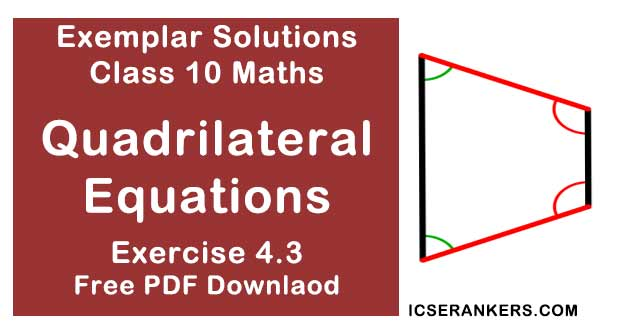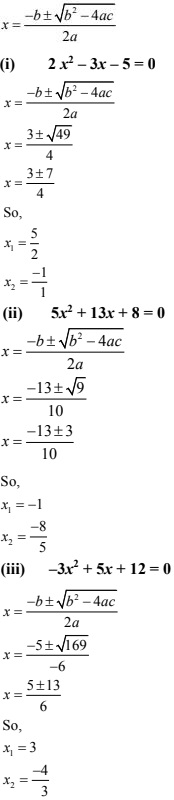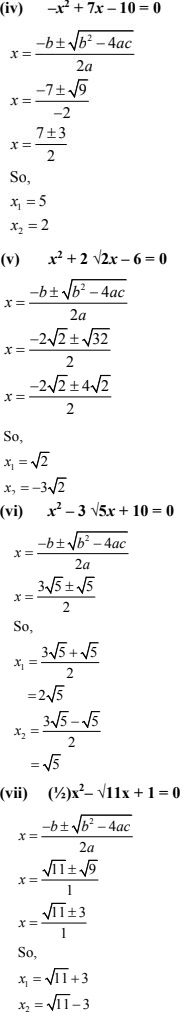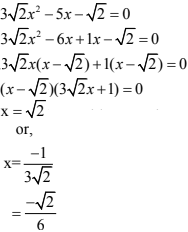# Chapter 4 Quadrilateral Equations NCERT Exemplar Solutions Exercise 4.3 Class 10 MathsChapter Name NCERT Maths Exemplar Solutions for Chapter 4 Quadrilateral Equations Exercise 4.3 Book Name NCERT Exemplar for Class 10 Maths Other Exercises Exercise 4.1Exercise 4.2Exercise 4.4 Related Study NCERT Solutions for Class 10 Maths

### Exercise 4.3 Solutions

1. Find the roots of the quadratic equations by using the quadratic formula in each of the following.
(i) 2x2 - 3x - 5 = 0
(ii) 5x2 + 13x + 8 = 0
(iii) -3x2 + 5x + 12 = 0
(iv) -x2 + 7x - 10 = 0
(v) x2 + 2√2x - 6 = 0
(vi) x2 - 3√5x + 10 = 0
(vii) (1/2)x2 - √11x + 1 = 0

Solution
The quadratic formula to find the roots of quadratic equation ax2 + bx + c = 0, a ≠ 0 is given by,2. Find the roots of the following quadratic equations by the factorization method :
(i) 2x2 + (5/3)x - 2 = 0
(ii) (2/5)x2 - x - 3/5 = 0
(iii) 3√2 x2 - 5x - √2 = 0
(iv) 3x2 + 5√5x - 10 = 0
(v) 21x2 - 2x + 1/21 = 0
Solution
(i) 2x2 + (5/3)x - 2 = 0
⇒ 6x2 + 5x - 6 = 0
⇒ 6x2 + 9x - 4x - 6 = 0
⇒ 3x(2x + 3)-2(2x + 3) = 0
⇒ (2x + 3)(3x - 2) = 0
⇒ 2x + 3  = 0 or 3x - 2 = 0
⇒ 2x = -3 or 3x = 2
So, the roots of the given quadratic equation are -3/2 and 2/3.

(ii) (2/5)x2 - x - 3/5 = 0
⇒ 2x2 - 5x - 3 = 0
⇒ 2x2 - 6x + 1x - 3 = 0
⇒ 2x(x - 3) + 1(x - 3) = 0
⇒ (x - 3)(2x + 1) = 0
⇒ x - 3 = 0
and,
2x + 1 = 0
⇒ x = 3
or 2x = -1
So, the roots of the quadratic equation are 3 and -1/2.

(iii)(iv) 3x2 + 5√5x - 10 = 0
⇒ 3x2 + 5√5x - 10 = 0
⇒ 3x2 + 6√5x - √5 - 10 = 0
⇒ 3x(x + 2√5) - √5(x + 2√5) = 0
⇒ (x + 2√5)(3x - √5) = 0
⇒ x = √5/3
or x = -2√5
So, these are the roots of the given quadratic equation.

(v) 21x2 - 2x + 1/21 = 0
⇒ 441x2 - 42x + 1 = 0
⇒ 441x2 - 21x - 21x + 1 = 0
⇒ 21x( 21x - 1) - 1(21x - 1) = 0
⇒ (21x - 1)(21x - 1) = 0
⇒ (21x - 1) = 0 or (21x - 1) = 0
⇒ 21x = 1 or 21x = 1
⇒ x = 1/21, 1/21
So, the roots of the given equation are x = 1/21, 1/21